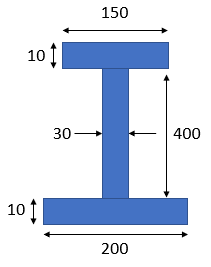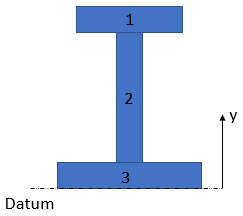## Beam Centroid

Calculate the centroid for the I-Beam cross section below.Hint
We can determine the centroid by breaking down the cross section into three segments, and setting a datum at the beam's bottom face (you could easily set the datum elsewhere like the top, or broken the segments into different areas):Hint 2
To find the centroid:
$$\bar{y}=\frac{\sum A_iy_i}{\sum A_i}$$$where $$A_i$$ is the individual segment's area, and $$y_i$$ is the individual segment's centroid distance from a reference line or datum. We can determine the centroid by breaking down the cross section into three segments, and setting a datum at the beam's bottom face (you could easily set the datum elsewhere like the top, or broken the segments into different areas):To find the centroid, we use the formula: $$\bar{y}=\frac{\sum A_iy_i}{\sum A_i}$$$
where $$A_i$$ is the individual segment's area, and $$y_i$$ is the individual segment's centroid distance from a reference line or datum.

Segment 1:
$$A_1=150\times 10=1500$$$$$y_1=10+400+\frac{10}{2}=415$$$
Segment 2:
$$A_2=30\times 400=12,000$$$$$y_2=10+\frac{400}{2}=210$$$
Segment 3:
$$A_3=10\times 200=2000$$$$$y_3=\frac{10}{2}=5$$$

Thus,
$$\bar{y}=\frac{A_1y_1+A_2y_2+A_3y_3}{A_1+A_2+A_3}$$$$$\bar{y}=\frac{(1,500)(415)+(12,000)(210)+(2,000)(5)}{(1,500+12,000+2,000)}$$$
$$\bar{y}=\frac{622,500+2,520,000+10,000}{15,500}=\frac{3,152,500}{15,500}=203.4$$\$
203.4